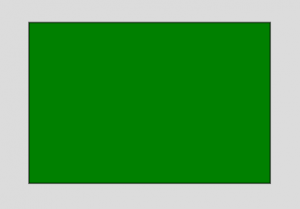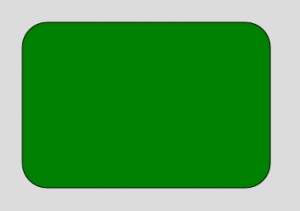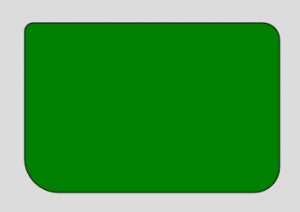# p5.js | rect() Function

The rect() function is an inbuilt function in p5.js which is used to draw the rectangle on the screen. A rectangle contains four sides and four angles. Each angle of the rectangle is 90 degree. The length of the opposite sides of rectangles are equal.

Syntax:

`rect( x, y, w, h, tl, tr, br, bl )`

or

`rect( x, y, w, h, detailX, detailY ) `

Parameters: This function accepts many parameters as mentioned above and descibed below:

• x: It is used to set the x-coordinate of rectangle.
• y: It is used to set the y-coordinate of rectangle.
• w: It is used to set the width of rectangle.
• h: It is used to set the height of rectangle.
• tl: It is optional parameter and used to set the radius of top-left corner.
• tr: It is optional parameter and used to set the radius of top-right corner.
• br: It is optional parameter and used to set the radius of bottom-right corner.
• bl: It is optional parameter and used to set the radius of bottom-left corner.
• detailX: It is used to set the number of segment in x-direction.
• detailY: It is used to set the number of segment in y-direction.

Example 1:

 `function` `setup() {  ` `      `  `    ``// Create Canvas of given size  ` `    ``createCanvas(400, 300);  ` `  `  `}  ` `  `  `function` `draw() {  ` `      `  `    ``background(220); ` `      `  `    ``// Use color() function ` `    ``let c = color(``'green'``); ` `  `  `    ``// Use fill() function to fill color ` `    ``fill(c); ` `    `  `    ``// Draw a rectangle ` `    ``rect(50, 50, 300, 200); ` `    `  `}  `

Output:Example 2:

 `function` `setup() {  ` `      `  `    ``// Create Canvas of given size  ` `    ``createCanvas(400, 300);  ` `  `  `}  ` `  `  `function` `draw() {  ` `      `  `    ``background(220); ` `      `  `    ``// Use color() function ` `    ``let c = color(``'green'``); ` `  `  `    ``// Use fill() function to fill color ` `    ``fill(c); ` `    `  `    ``// Draw a rectangle ` `    ``rect(50, 50, 300, 200, 30); ` `    `  `}  `

Output:Example 3:

 `function` `setup() {  ` `      `  `    ``// Create Canvas of given size  ` `    ``createCanvas(400, 300);  ` `  `  `}  ` `  `  `function` `draw() {  ` `      `  `    ``background(220); ` `      `  `    ``// Use color() function ` `    ``let c = color(``'green'``); ` `  `  `    ``// Use fill() function to fill color ` `    ``fill(c); ` `    `  `    ``// Draw a rectangle  ` `    ``rect(50, 50, 300, 200, 10, 20, 30, 40); ` `    `  `}  `

Output:Online editor: https://editor.p5js.org/
Environment Setup: https://www.geeksforgeeks.org/p5-js-soundfile-object-installation-and-methods/

Reference: https://p5js.org/reference/#/p5/rectMy Personal Notes arrow_drop_upCheck out this Author's contributed articles.

If you like GeeksforGeeks and would like to contribute, you can also write an article using contribute.geeksforgeeks.org or mail your article to contribute@geeksforgeeks.org. See your article appearing on the GeeksforGeeks main page and help other Geeks.

Please Improve this article if you find anything incorrect by clicking on the "Improve Article" button below.

Article Tags :

Be the First to upvote.

Please write to us at contribute@geeksforgeeks.org to report any issue with the above content.